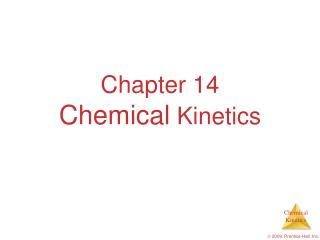DownloadDownload PresentationChapter 14 Chemical Kinetics

# Chapter 14 Chemical Kinetics

Download Presentation## Chapter 14 Chemical Kinetics

- - - - - - - - - - - - - - - - - - - - - - - - - - - E N D - - - - - - - - - - - - - - - - - - - - - - - - - - -
##### Presentation Transcript

1. Chapter 14Chemical Kinetics  2009, Prentice-Hall, Inc.

2. Reaction Coordinate Diagrams It is helpful to visualize energy changes throughout a process on a reaction coordinate diagram like this one for the rearrangement of methyl isonitrile.  2009, Prentice-Hall, Inc.

3. Reaction Coordinate Diagrams • The diagram shows the energy of the reactants and products (and, therefore, E). • The high point on the diagram is the transition state. • The species present at the transition state is called the activated complex. • The energy gap between the reactants and the activated complex is the activation energy barrier.  2009, Prentice-Hall, Inc.

4. Maxwell–Boltzmann Distributions • Temperature is defined as a measure of the average kinetic energy of the molecules in a sample. • At any temperature there is a wide distribution of kinetic energies.  2009, Prentice-Hall, Inc.

5. Maxwell–Boltzmann Distributions • As the temperature increases, the curve flattens and broadens. • Thus at higher temperatures, a larger population of molecules has higher energy.  2009, Prentice-Hall, Inc.

6. Maxwell–Boltzmann Distributions • If the dotted line represents the activation energy, then as the temperature increases, so does the fraction of molecules that can overcome the activation energy barrier. • As a result, the reaction rate increases.  2009, Prentice-Hall, Inc.

7. -Ea RT Maxwell–Boltzmann Distributions This fraction of molecules can be found through the expression where R is the gas constant and T is the Kelvin temperature. f = e  2009, Prentice-Hall, Inc.

8. -Ea RT Arrhenius Equation Svante Arrhenius developed a mathematical relationship between k and Ea: k = A e where A is the frequency factor, a number that represents the likelihood that collisions would occur with the proper orientation for reaction.  2009, Prentice-Hall, Inc.

9. Ea R 1 T 1 T Arrhenius Equation Taking the natural logarithm of both sides, the equation becomes ln k = - () + ln A y = m x + b Therefore, if k is determined experimentally at several temperatures, Eacan be calculated from the slope of a plot of ln k vs. .  2009, Prentice-Hall, Inc.

10. Reaction Mechanisms The sequence of events that describes the actual process by which reactants become products is called the reaction mechanism.  2009, Prentice-Hall, Inc.

11. Reaction Mechanisms • Reactions may occur all at once or through several discrete steps. • Each of these processes is known as an elementary reaction or elementary process.  2009, Prentice-Hall, Inc.

12. Reaction Mechanisms The molecularity of a process tells how many molecules are involved in the process.  2009, Prentice-Hall, Inc.

13. Multistep Mechanisms • In a multistep process, one of the steps will be slower than all others. • The overall reaction cannot occur faster than this slowest, rate-determining step.  2009, Prentice-Hall, Inc.

14. Slow Initial Step NO2(g) + CO (g) NO (g) + CO2(g) • The rate law for this reaction is found experimentally to be Rate = k [NO2]2 • CO is necessary for this reaction to occur, but the rate of the reaction does not depend on its concentration. • This suggests the reaction occurs in two steps.  2009, Prentice-Hall, Inc.

15. Slow Initial Step • A proposed mechanism for this reaction is Step 1: NO2 + NO2 NO3 + NO (slow) Step 2: NO3 + CO  NO2 + CO2 (fast) • The NO3 intermediate is consumed in the second step. • As CO is not involved in the slow, rate-determining step, it does not appear in the rate law.  2009, Prentice-Hall, Inc.

16. Fast Initial Step 2 NO (g) + Br2(g) 2 NOBr (g) • The rate law for this reaction is found to be Rate = k [NO]2 [Br2] • Because termolecular processes are rare, this rate law suggests a two-step mechanism.  2009, Prentice-Hall, Inc.

17. Step 1: NO + Br2 NOBr2 (fast) Fast Initial Step • A proposed mechanism is Step 2: NOBr2 + NO  2 NOBr (slow) Step 1 includes the forward and reverse reactions.  2009, Prentice-Hall, Inc.

18. Fast Initial Step • The rate of the overall reaction depends upon the rate of the slow step. • The rate law for that step would be Rate = k2 [NOBr2] [NO] • But how can we find [NOBr2]?  2009, Prentice-Hall, Inc.

19. Fast Initial Step • NOBr2 can react two ways: • With NO to form NOBr • By decomposition to reform NO and Br2 • The reactants and products of the first step are in equilibrium with each other. • Therefore, Ratef = Rater  2009, Prentice-Hall, Inc.

20. k1 k−1 [NO] [Br2] = [NOBr2] Fast Initial Step • Because Ratef = Rater , k1 [NO] [Br2] = k−1 [NOBr2] • Solving for [NOBr2] gives us  2009, Prentice-Hall, Inc.

21. Rate = [NO] [Br2] [NO] k2k1 k−1 Fast Initial Step Substituting this expression for [NOBr2] in the rate law for the rate-determining step gives = k [NO]2 [Br2]  2009, Prentice-Hall, Inc.

22. Catalysts • Catalysts increase the rate of a reaction by decreasing the activation energy of the reaction. • Catalysts change the mechanism by which the process occurs.  2009, Prentice-Hall, Inc.

23. Catalysts One way a catalyst can speed up a reaction is by holding the reactants together and helping bonds to break.  2009, Prentice-Hall, Inc.

24. Enzymes • Enzymes are catalysts in biological systems. • The substrate fits into the active site of the enzyme much like a key fits into a lock.  2009, Prentice-Hall, Inc.